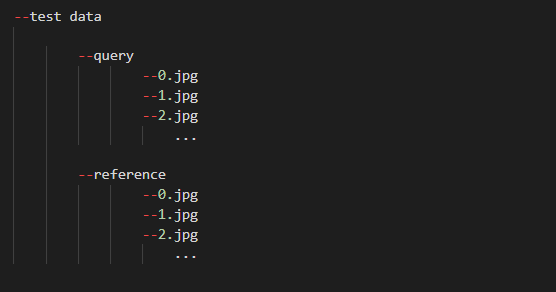# Question about bringing specific class folder in torchvision.ImageFolder

say there is directory , and there are query, reference folder inside test data directory.
what I want to do is make different dataset_loader for each query and reference images.
Need advice on this work. thanksI think the easiest way would be to write a custom `Dataset` and just load all images from the specified folder.
Here is a dummy snippet you could adapt to your use case:

``````class MyDataset(Dataset):
def __init__(self, path, transform=None):
self.image_paths = glob.glob(os.path.join(path, '*.jpg'))
self.transform = transform

def __getitem__(self, index):
x = Image.open(self.image_paths[index])
if self.transform:
x = self.transform(x)
return x

def __len__(self):
return len(self.image_paths)

query_folder = 'QUERY_PATH'
reference_folder = 'REFERENCE_PATH'
dataset_query = MyDataset(query_folder, transform=transforms.ToTensor())
dataset_reference = MyDataset(reference_folder, transform=transforms.ToTensor())
``````